# High School Physics : Understanding the Concept of Waves

## Example Questions

### Example Question #1 : Waves, Sound, And Light

Two waves, each with an amplitude of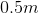are superimposed with constructive interference such that they are in phase. What is the resultant amplitude?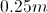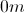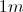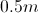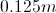Explanation:

When two waves are superimposed, the interference can either be constructive or destructive. In this case, the interference is constructive and the waves are in phase, which means we add the amplitudes together.

Since each wave has an amplitude of, our new amplitude will be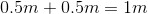.

### Example Question #1 : Waves, Sound, And Light

Two waves, one with an amplitude of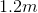and the other of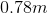are superimposed with destructive interference. What is the resultant amplitude?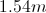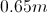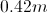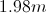Explanation:

When two waves are superimposed, the interference can be either constructive or destructive. In this case the interference is destructive, which means our resultant amplitude will be the difference of the two given amplitudes.

That means our new amplitude will be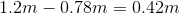.

### Example Question #1 : Understanding The Concept Of Waves

Two identical waves are superimposed with destructive interference. What will happen to the resulting wave?

It will be twice as large as each original wave.

It will be half as large as each original wave.

It will be still. There will be no wave.

The two waves will pass through each other without problem.